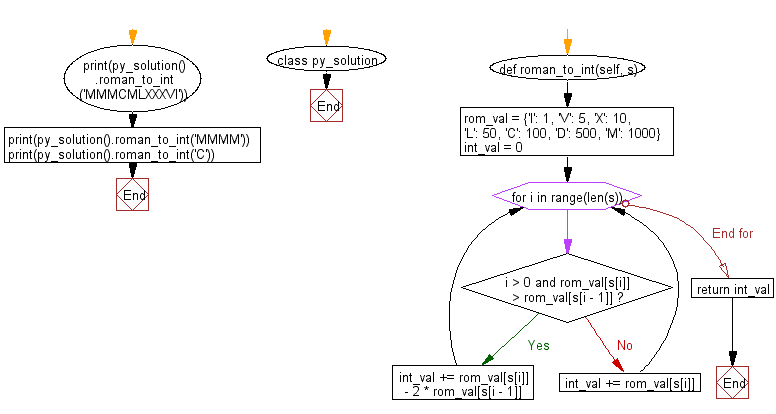﻿ Python: Convert a roman numeral to an integer - w3resource# Python: Convert a roman numeral to an integer

## Python Class: Exercise-2 with Solution

Write a Python class to convert a roman numeral to an integer.

Sample Solution:

Python Code:

``````class py_solution:
def roman_to_int(self, s):
rom_val = {'I': 1, 'V': 5, 'X': 10, 'L': 50, 'C': 100, 'D': 500, 'M': 1000}
int_val = 0
for i in range(len(s)):
if i > 0 and rom_val[s[i]] > rom_val[s[i - 1]]:
int_val += rom_val[s[i]] - 2 * rom_val[s[i - 1]]
else:
int_val += rom_val[s[i]]
return int_val

print(py_solution().roman_to_int('MMMCMLXXXVI'))
print(py_solution().roman_to_int('MMMM'))
print(py_solution().roman_to_int('C'))
```
```

Sample Output:

```3986
4000
100
```

Pictorial Presentation:Flowchart:## Visualize Python code execution:

The following tool visualize what the computer is doing step-by-step as it executes the said program:

Python Code Editor:

What is the difficulty level of this exercise?

Test your Programming skills with w3resource's quiz.

﻿

## Python: Tips of the Day

Join Strings in an Iterable:

```>>> words = ('Hello', 'Python', 'Programmers')
>>> '!'.join(words)
'Hello!Python!Programmers'
>>> words_dict = {0: 'zero', 1: 'one', 2: 'two', 3: 'three'}
>>> '&'.join(words_dict.values())
'zero&one&two&three'
```